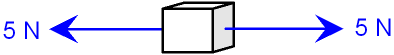gcsescience.com                                       14                                       gcsescience.com

Forces and Motion

Newton's First Law of Motion - Balanced Forces.

What is a Force?

A force is a push or a pull. It is measured in Newtons (N).

What are Balanced Forces?

Forces are balanced when the resultant force is zero.
If all of the forces which act on an object
along the same straight line are equal and opposite,
then when you subtract one side from the other the
resultant force is zero and the forces are called balanced.

What is a Free-Body Force Diagram?

The picture below is called a free-body force diagram.
It shows only the object and the forces acting on it.
The arrow shows the direction of the force and the
length of the arrow shows the size of the force (see vectors).The cube in the picture has two forces pulling in opposite directions.
The resultant force is 5 - 5 Newtons = 0 N. The forces are balanced.

What does Newton's First Law mean?

Newton's First Law says that if the
forces on an object are balanced then it will carry on as it is.

This means that
1. if the object is not moving then it will continue to stay still.
2. if the object is already moving
then it will continue to move with a constant velocity.

Number 2 goes against common sense.
We know that in the "real world" things slow down
and stop if there is "no force" to keep them moving.
Newton understood that there is an opposing force
which makes things slow down and stop. It is called friction.

gcsescience.com          Physics Quiz          Index          Force Quiz          gcsescience.com